GMAT  >  Test: Rectangles And Squares- 2

# Test: Rectangles And Squares- 2

Test Description

## 20 Questions MCQ Test Quantitative for GMAT | Test: Rectangles And Squares- 2

Test: Rectangles And Squares- 2 for GMAT 2023 is part of Quantitative for GMAT preparation. The Test: Rectangles And Squares- 2 questions and answers have been prepared according to the GMAT exam syllabus.The Test: Rectangles And Squares- 2 MCQs are made for GMAT 2023 Exam. Find important definitions, questions, notes, meanings, examples, exercises, MCQs and online tests for Test: Rectangles And Squares- 2 below.
Solutions of Test: Rectangles And Squares- 2 questions in English are available as part of our Quantitative for GMAT for GMAT & Test: Rectangles And Squares- 2 solutions in Hindi for Quantitative for GMAT course. Download more important topics, notes, lectures and mock test series for GMAT Exam by signing up for free. Attempt Test: Rectangles And Squares- 2 | 20 questions in 40 minutes | Mock test for GMAT preparation | Free important questions MCQ to study Quantitative for GMAT for GMAT Exam | Download free PDF with solutions
 1 Crore+ students have signed up on EduRev. Have you?
Test: Rectangles And Squares- 2 - Question 1

###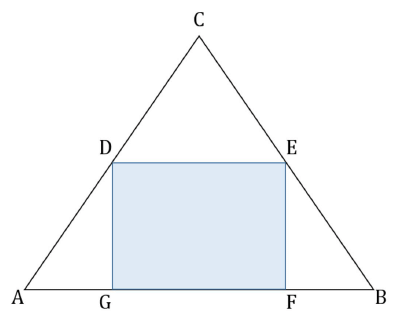Rectangle DEFG is inscribed in equilateral triangle as shown above. What is the area of triangle ABC? (1) The area of rectangle DEFG is 8√3 square units (2) The area of triangle AGD is 2√3 square units

Detailed Solution for Test: Rectangles And Squares- 2 - Question 1

Step 1 & 2: Understand Question and Draw Inference

Given

• ΔABC is equilateral
• ∠ABC = ∠BAC = ∠ACB = 60°
• Points D, E, F, G lie on the triangle
• DEFG is a rectangle
• Each ∠ of this rectangle is 90°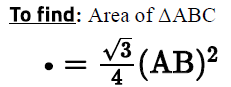• To find the area, we need to find AB
• Let’s represent the relation between different sides and ∠s of the figure:
• ∠CDE = ∠ CAB = 60° (corresponding ∠s)
• (Since the opposite sides of a rectangle are parallel, DE || GF, and therefore, DE || AB)
• Similarly, ∠ CED = ∠ CBA = 60°.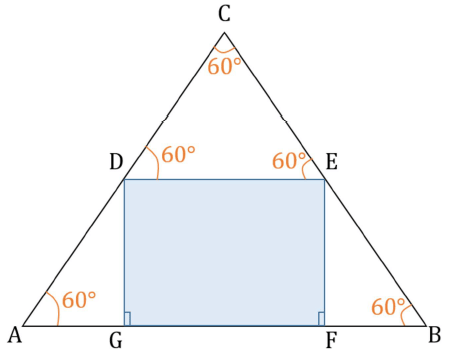• Assuming the sides of the rectangle:
• GF = x units
• GD = EF = y units.
• Expressing all the unknown lengths in terms of x and y:
• In equilateral ΔDEC, CD = CE = side DE of the rectangle = x
• ΔEFB is a 30-60-90 triangle
• Hence we can write : FB : EF : EB = 1 : √3 : 2

​​​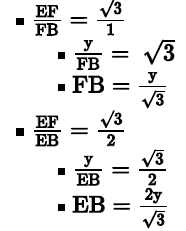• Exactly corresponding calculations will also apply in right ΔDGA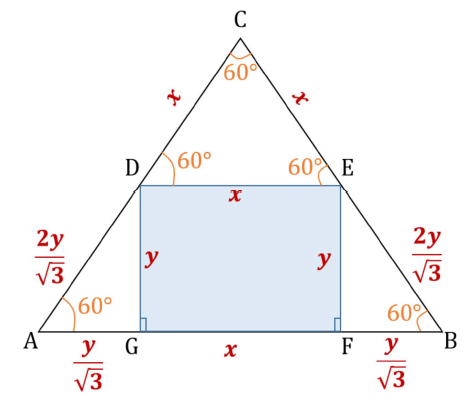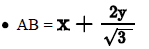Thus, in order to find the area of the triangle, we need to know the value of x and y.

Step 3 : Analyze Statement 1 independent

• The area of rectangle DEFG is 8√3 square units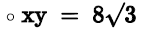• Multiple values of x and y will satisfy this equation.
• Hence, Statement 1 is not sufficient.

Step 4 : Analyze Statement 2 independent

• The area of triangle AGD is 2√3 square units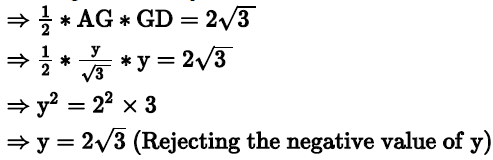• But we don’t know the value of x.
• Hence, Statement 2 is also not sufficient.

Step 5: Analyze Both Statements Together (if needed)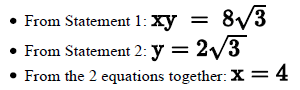• Since we now know the value of x and y, we can find the value of AB.
• Hence, by combining both the statement we can get our answer.

Therefore, the correct answer is Option C

Test: Rectangles And Squares- 2 - Question 2

###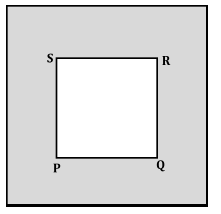A frame of uniform width is placed around a square photograph PQRS. If PQ = 10 inches and the area of the frame is 125 square inches, what is the width of the frame, in inches?

Detailed Solution for Test: Rectangles And Squares- 2 - Question 2

Given:

• A square PQRS, whose side length = PQ = 10 inches
• A frame of uniform width, say w
• Let’s label the outer boundary of the frame as ABCD
• So, the given information can be represented visually as under: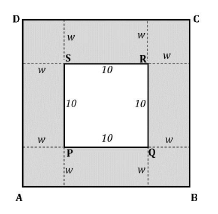• From the figure, it is easy to see that AB = BC = CD = DA = 10 + 2w
• Therefore, ABCD is a square as well
• Area of frame = 125 square inches

To find: w = ?
Approach:

1. To find w, we first need to develop a relation in w
2. (Area of frame) = (Area of outer square ABCD) – (Area of inner square PQRS)

•  We know the Area of frame
• We can easily calculate (Area of inner square PQRS)
• Since we’ve expressed the side length of square ABCD in terms of w, we’ll get the expression for (Area of outer square ABCD) in terms of w
d. So, from the above equation, we can find the value of w

Working Out
(Area of frame) = (Area of outer square ABCD) – (Area of inner square PQRS)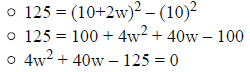At this point, a way to split the middle term 40w into factors doesn’t immediately suggest itself to us. Therefore, we will solve the equation by the method of ‘completing the square’ instead. In this method, we will try to express the quadratic expression in the left hand side of the equation in the form a2 +2ab + b2 , so that we can then write it as (a+b) . Look below.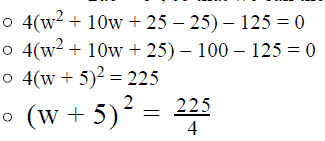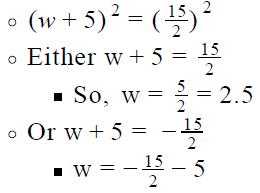• This value of w is rejected because the width of a frame cannot be negative
• Therefore, w = 2.5

Test: Rectangles And Squares- 2 - Question 3

### The rectangle ABCD has its side lengths equal to x and y respectively, where x and y are prime numbers greater than 2. Which of the following cannot be equal to the sum of all the sides of the rectangle ABCD? I. 64 II. 82 III. 146

Detailed Solution for Test: Rectangles And Squares- 2 - Question 3

Given

• ABCD is a rectangle with side lengths equal to x and y respectively
• x, y are prime numbers > 2
• Thus, x and y are odd

To Find

• Values in the options that cannot be equal to 2(x+y)

Approach and Working

• As we are asked to find a value in the options that is not equal to 2(x+y), we will first find a constraint on 2(x+y).
• We will then evaluate the 3 options as per the constraint on 2(x+y)
• Since x and y are both odd, x + y = even, i.e. it will at least be a multiple of 2, if not its higher powers
• So, 2(x+y) will at least be a multiple of 4, if not the higher powers of 2
• Hence an integer that is not divisible by 4 can never be equal to 2(x+y)

1. Option-I: 64 → Is divisible by 4, can be equal to 2(x+y)
2. Option-II: 82 → Is not divisible by 4, can never be equal to 2(x+y)
3. Option-III: 146 → Is not divisible by 4, can never be equal to 2(x+y)
Thus, options II and III can never be equal to the value of 2(x+y).
Hence the correct answer is OPTION E

Test: Rectangles And Squares- 2 - Question 4

A wire was initially used to fence a right-triangular plot of land along its perimeter. The lengths, in metres, of the perpendicular sides of the plot were both integers and in the ratio 3:4. The wire was later removed from the triangular plot and used to fence a square plot along its perimeter. When the square plot had been completely fenced, 4 metres of wire still remained. Which of the following could represent the greatest distance between two points in the square plot?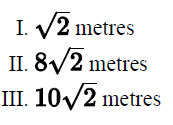Detailed Solution for Test: Rectangles And Squares- 2 - Question 4

Given

• Let’s assume the perpendicular sides of the right triangular plot as 3x and 4x meters (both given to be integers)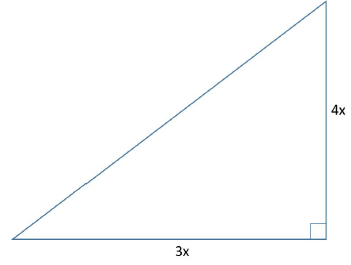• Let the side of the square plot be s meters
• Initial length of wire, w = (Perimeter of Square) + 4 meters
• w = 4s + 4

To Find: Which of the 3 values could be the greatest distance between two points in the square plot?

Approach:

• The greatest distance between two points in the square plot, say D = Length of the diagonal of the square plot =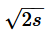• So, in order to find the length of the diagonal, we need to know s.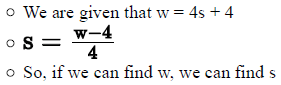• Now, w = (Perimeter of the right triangle whose perpendicular sides are3x and 4x). Using this fact, we’ll find the value of w in x. Using this value, we’ll be able to find the length of diagonal in terms of x.
• Now remember the given constraint that 3x and 4x must be integers. We’ll use this constraint to evaluate the 3 options.

Working out

1. Applying Pythagoras Theorem in the right triangle, we get: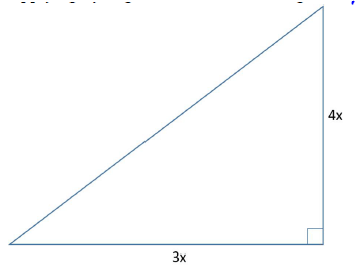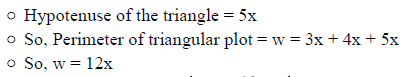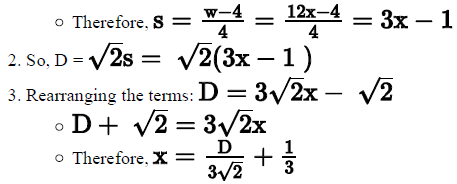4. We will now check the 3 given possible lengths of the diagonal to see if they lead to integral values of 3x and 4x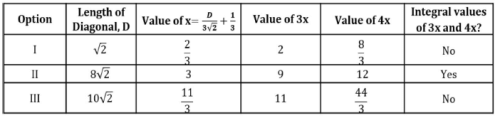• Thus, only Option II satisfies the constraint that 3x and 4x must be integers.

Looking at the answer choices, we see that the correct answer is Option B

Test: Rectangles And Squares- 2 - Question 5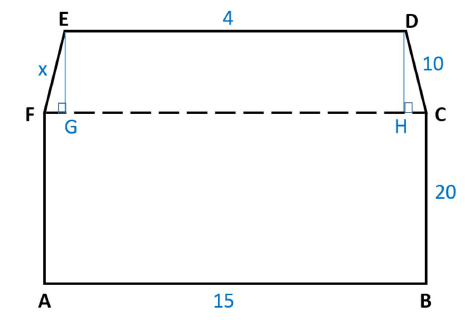A student initially draws a rectangle ABCF. Later, he draws an adjacent trapezium FCDE in which side ED is parallel to side FC and drops perpendiculars EG and DH on FC. The dimensions of each side of the polygon ABCDEF, in centimeters, are shown on the figure above. What is the value of x?
(1) The area of the polygon ABCHDEG is 332 square centimeters
(2) If sides AE and BD are joined, the area of the quadrilateral ABDE will be 266 square centimeters

Detailed Solution for Test: Rectangles And Squares- 2 - Question 5

Step 1 & 2: Understand Question and Draw Inference

Given

• Rectangle ABCF
• Since opposite sides of a rectangle are equal,
• CF = AB = 15 cm and
• AF = BC = 20 cm
• Trapezium EDCF
• ED parallel to FC
• CF = 15 cm
• ED = 4 cm
• CD = 10 cm

To find: x = ?

Step 3 : Analyze Statement 1 independent

• The area of the polygon ABCHDEG is 332 square centimeters
• To make use of this fact, let’s first try to understand how the area of this figure will be found.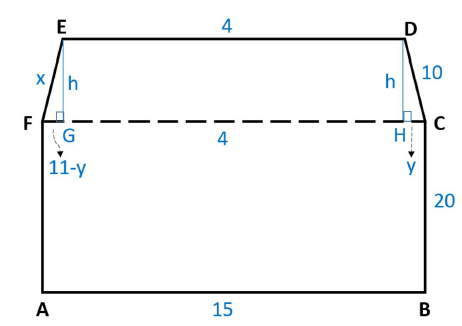• Area of the figure
• = area of rectangle EDHG + area of rectangle ABCF
• Since ED is parallel to FC, both perpendiculars EG and DH will have the same length. Let this length be h.
• Let CH = y.
• Since EDHG forms a rectangle, GH = 4
• So, FG = FC – (GH + HC)
• That is, FG = 15 – (4 + y)
• FG = 11 – y
• So, area of the figure = 4h + 20 * 15
• Substituting the value of area in the above equation: 4h + 20 * 15 = 332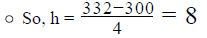• Also, by applying Pythagoras Theorem in right triangle DHC,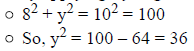• So, y = 6 (rejecting the negative value as length cannot be negative)
• And, in right triangle EHF, we get:
• (11-y)2+ h2 = x2
• Substituting y = 6 and h = 8 in this equation, we get:
• 52 + 82 = x2
• That is, x2 = 89
• So, x = √89 (rejecting the negative value since length cannot be negative)
Thus, Statement 1 is sufficient to determine the length of x.

Step 4 : Analyze Statement 2 independent

• If sides AE and BD are joined, the area of the quadrilateral ABDE will be 266 square centimeters
• In quadrilateral ABDE, sides AB and ED are parallel (since both ED and AB are parallel to FC)
• This means, ABDE is a trapezium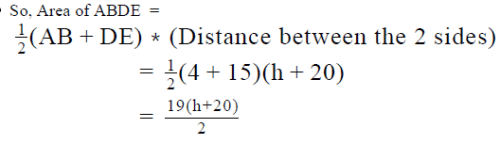• Substituting the known value of this area in the above equation: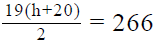• Since this is a linear equation with only one unknown, we can use it to find a unique value of h.
• Once we know the value of h, we can find the value of x using similar analysis as done in Statement 1 above.

So, Statement 2 is sufficient to determine a unique value of x.

Step 5: Analyze Both Statements Together (if needed)

Since we’ve already arrived at unique answers in each of Steps 3 and 4, this
step is not required

Test: Rectangles And Squares- 2 - Question 6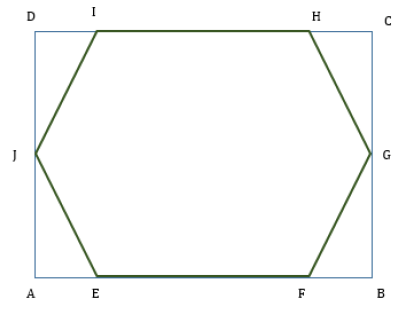In the figure above, a regular hexagon EFGHIJ is inscribed in the rectangle ABCD. If the ratio of the magnitudes of the area and the perimeter of the hexagon is √3/2 , what is the ratio of the magnitudes of the area and the perimeter of the rectangle ABCD?

Detailed Solution for Test: Rectangles And Squares- 2 - Question 6

Given

• Rectangle ABCD
• Let the length and breadth be l and b respectively
• Regular hexagon EFGHIJ
• Let the side length be x
•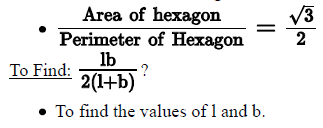Approach

1. For finding the values of l and b, we will try to express l and b in terms of the length of the side of the hexagon, i.e. x.
1. As we are given the ratio of the area and perimeter of the hexagon, we will use this relation to find the value of x
2. Area of hexagon = 6 * Area of Equilateral Triangle with length x
3. Perimeter of hexagon = 6x
2. As the hexagon is inside the rectangle, we can express l and b in terms of x.
1. Since it is a regular hexagon, we can calculate its interior angle using the formula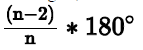2. Using this information, we can calculate all the angles of the corner triangles.
3. The above analysis will help us express l and b in terms of x

Working out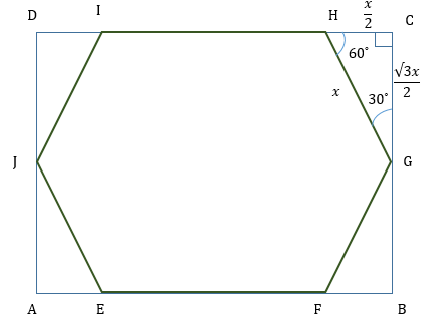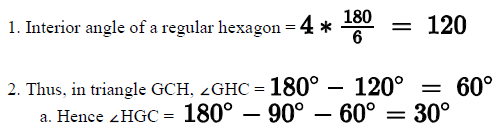b. As it is a  30o-60o-90triangle, with the hypotenuse being equal to x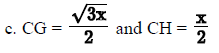3. All the 3 other triangles will have the same side lengths and angles as triangle GCH.
4. So, we can write length of the rectangle, l = CD = CH + HI + ID =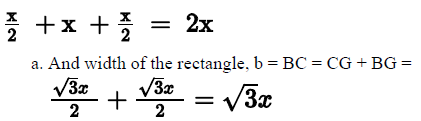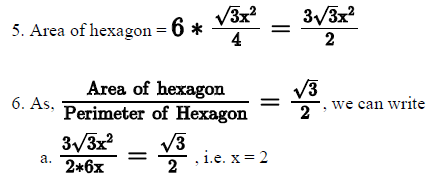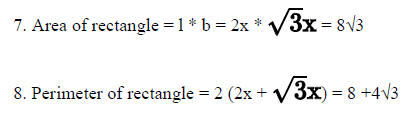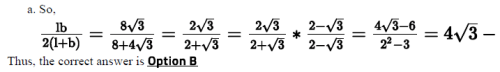Test: Rectangles And Squares- 2 - Question 7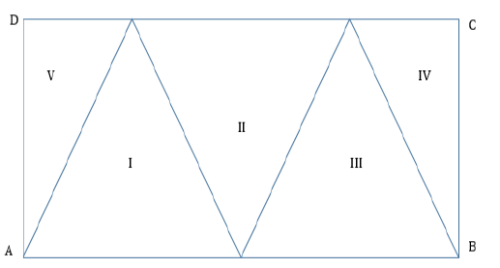In the figure given above, ABCD is a rectangle that is divided into five triangular regions. If triangles I, II and III have equal area and triangles IV and V have equal area, what is the area of rectangle  ABCD?
(1) The area of triangle I is 30
(2) The area of triangle V is 15

Detailed Solution for Test: Rectangles And Squares- 2 - Question 7

Step 1 & 2: Understand Question and Draw Inference

• Rectangle ABCD
• Triangles I, II and III have same areas
• As they lie between the same set of parallel lines, their height (h) would be equal
• So, their base would also be equal
• AB = AE + EB (but AE= EB), let’s assume AE=EB =x
• So, AB = 2x
• Similarly, base of triangle II = FG = x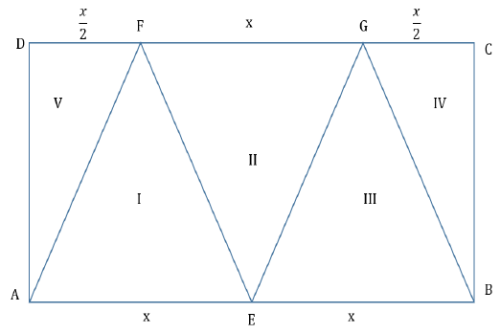• Triangles IV and V have the same areas.
• As triangles IV and V lie between the same parallel line, their height (h) would be equal
• So, their bases would also be equal
• CD = AB = 2x
• DF + FG + GC = 2x
• 2DF + x= 2x
• DF = GC =

To Find: Area of rectangle ABCD = 2x * h

Step 3 : Analyze Statement 1 independent

(1) The area of triangle I is 30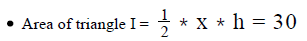As we know the value of x*h, we can find the value of area of rectangle ABCD.

Step 4 : Analyze Statement 2 independent

(2) The area of triangle V is 15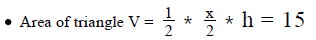As we know the value of x*h, we can find the value of area of rectangle
ABCD.

Step 5: Analyze Both Statements Together (if needed)

As we have a unique answer from steps 3 and 4, this step is not required.

Test: Rectangles And Squares- 2 - Question 8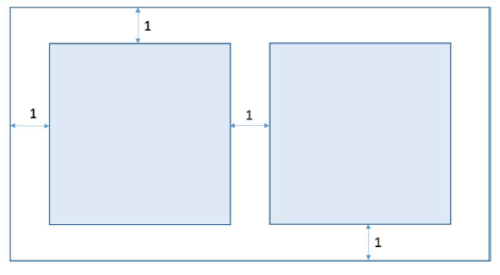Two identical square paintings are pasted on a rectangular wooden display board such that the edges of each painting are parallel to the corresponding edges of the display board and the two paintings are symmetrical about an imaginary line passing through the middle of the display board. The margins between the paintings and between the paintings and the board are as shown in the figure above. If the area of the display board is 66 square units, what is the area of each square painting?

Detailed Solution for Test: Rectangles And Squares- 2 - Question 8

Given

• Let the length and the breadth of the display board be L and B respectively.
• The area of the board = 66 square units
• LB = 66
• Let the side of each identical square painting be S
• So, L, B, S and the given margins can be shown in the figure as below: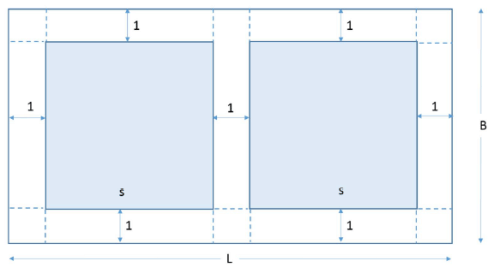To Find: The area of one square painting

Approach and working:

• Area of one square painting = S2

So, to answer the question, we need to find the value of S.

• To find the value of S, we need to draft an equation in terms of S. We’re given that LB = 66. So, if we can express L and B in terms of S, we will get an equation in terms of S.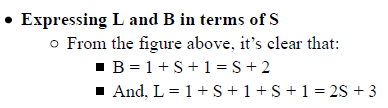Finding the value of S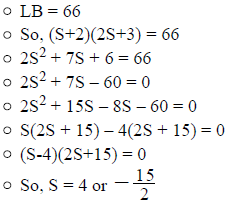• Rejecting the negative value since length cannot be negative.
• Therefore, S = 4
• Finding the value of required Area
• So, Area of one square painting = S2 = 42 = 16 square units

Looking at the answer choices, we see that the correct answer is Option D

Test: Rectangles And Squares- 2 - Question 9

A flowerbed of uniform width is developed around a rectangular patch of grass such that the outer length of the flowerbed is two-thirds more than the length of the rectangular patch and the outer breadth of the flowerbed is 100 percent greater than the breadth of the rectangular patch. If the area of the flowerbed is 8 square units more than twice the area of the rectangular patch, what is the area of the rectangular patch of grass?

Detailed Solution for Test: Rectangles And Squares- 2 - Question 9

Given:

Representing the given scenario visually.• Let the length of the rectangular patch of grass be Lp and the breadth be Bp
• Let the uniform width of the flowerbed be w
• And let the outer length of the flowerbed be LF and the breadth be BF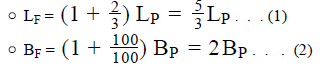• (Area of flower bed) = 2(Area of patch) + 8
• That is, (Area of outer rectangle – Area of patch) = 2(Area of patch) +8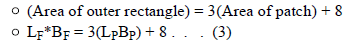To find : Area of the patch

Approach:

1.  Area of the patch = Lp *Bp
1. So, we need to find the value of L and B or at least of their product to be able to do this​
2. By using Equations (1), (2) and (3) together, we’ll be able to find the value of product Lp *Bp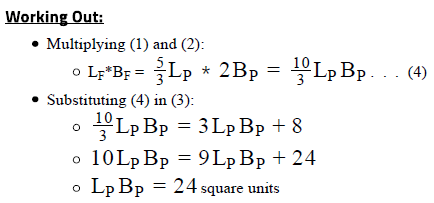Looking at the answer choices, we see that the correct answer is Option C

Test: Rectangles And Squares- 2 - Question 10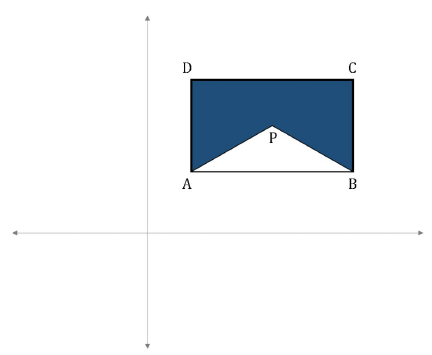In the xy-coordinate plane, polygon APBCDA is formed by placing rectangle ABCD and triangle APB as shown above. If sides AB and AD are parallel to the x- and the y- axes respectively, what is the area of polygon APBCDA?

(1) The coordinates of points A and C are (6, 8) and (12, 12) respectively
(2) The coordinates of point P are (8, 10)

Detailed Solution for Test: Rectangles And Squares- 2 - Question 10

Step 1 & 2: Understand Question and Draw Inference

Given: Rectangle ABCD and triangle APB in the xy-plane

• Since AB is parallel to the x-axis,
• Points A and B will have the same y-coordinates
• Since side CD of the rectangle will be parallel to side AB, points C and D too will have the same y-coordinates
• Since AD is parallel to the y-axis,
• Points A and D will have the same x-coordinates
• Since side BC of the rectangle will be parallel to side AD, points B and C too will have the same x-coordinates

To find: Area of polygon APBCDA

• = (Area of Rectangle ABCD) – (Area of triangle APB)
• Thus, in order to answer the question, we need to know the area of both Rectangle ABCD and triangle APB

Step 3 : Analyze Statement 1 independent

Statement 1 says that ‘The coordinates of points A and C are (6, 8) and (12, 12) respectively’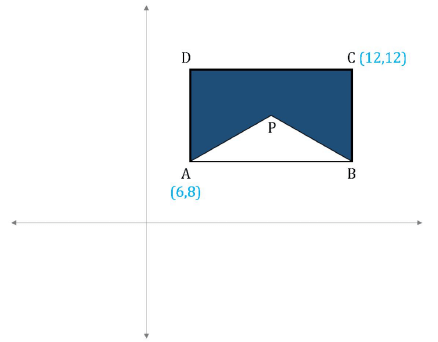• As discussed before, since AB and CD are parallel to the x-axis,
• (y-coordinate of point B) = (y-coordinate of point A) = 8
• (y-coordinate of point D) = (y-coordinate of point C) = 12
• Since AD and BC are parallel to the y-axis,
• (x-coordinate of point B) = (x-coordinate of point C) = 12
• (x-coordinate of point D) = (x-coordinate of point A) = 6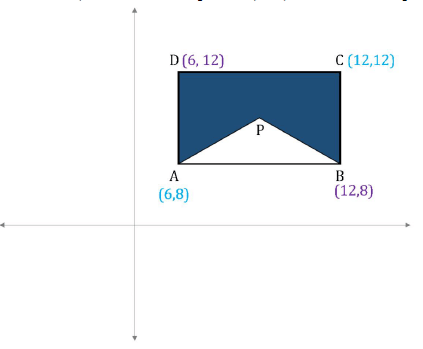• Therefore, AB = 12 – 6 = 6 units
• And BC = 12 – 8 = 4 units
• So, area of rectangle ABCD = 6*4 = 24 square units
• However, since Statement 1 doesn’t tell us about the area of triangle APB, it is not sufficient to answer the question.

Step 4 : Analyze Statement 2 independent

Statement 2 says that ‘The coordinates of point P are (8, 10)’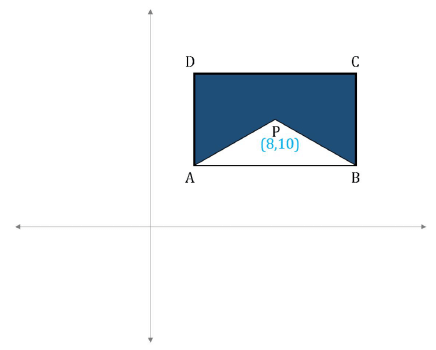From this statement, we can find neither the area of triangle APB nor the area of rectangle ABCD
So, this Statement is clearly insufficient.

Step 5: Analyze Both Statements Together (if needed)

• From Statement 1, we inferred that area of rectangle ABCD = 24 square units. We also came to know the coordinates of each of the points A, B, C and D
• From Statement 2, point P is (8, 10)
• Combining the 2 statements,
• The combined figure that we get is: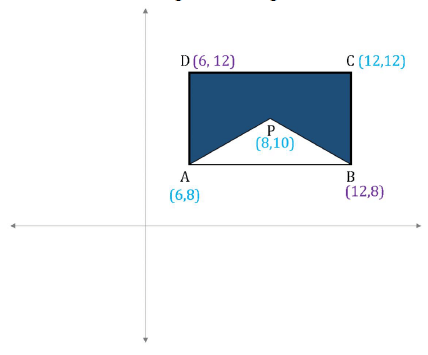• Dropping a perpendicular PQ from point P on side AB, we get: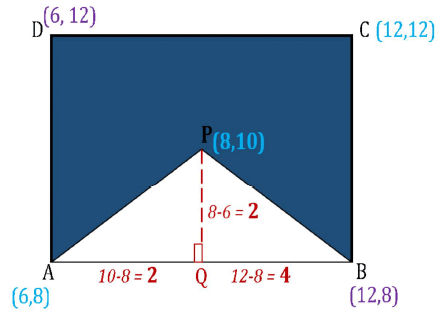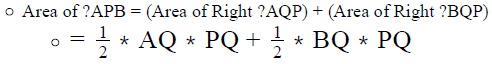• Since we know the coordinates of points A, P and B, we can find the lengths of sides AQ, PQ and BQ, as shown in the figure above
• Thus, by combining the 2 statements, we will be able to find the area of triangle APB
• Since we now know the area of both rectangle ABCD and the area of triangle APB, we can find the area of polygon APBCDA

So, the 2 statements together are sufficient to answer the question Answer: Option C

Test: Rectangles And Squares- 2 - Question 11

The length of a rectangle is 2 units greater than its breadth. If a diagonal of the rectangle measures 10 units, what is the perimeter of the rectangle?

Detailed Solution for Test: Rectangles And Squares- 2 - Question 11

Given

• Let the breadth of the rectangle be b units
• So, Length = b + 2 units
• Diagonal = 10 units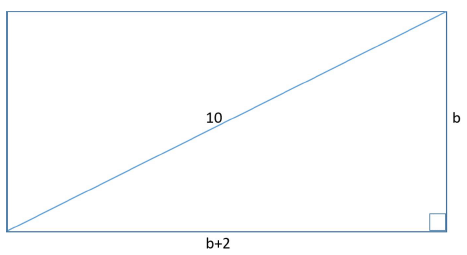To Find: Perimeter

Approach &  Working out

1. Perimeter of the rectangle = 2[b + (b+2)] = 2(2b+2) = 4(b+1)
2. So, to find the perimeter, we need to know the value of b
1. We’ll use Pythagoras Theorem on the diagonal, breadth and length of the rectangle to find the value of b
• Finding the value of b: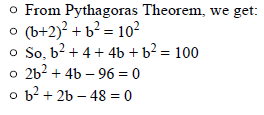• (b+8)(b-6) = 0
• b = 6 or -8
• Rejecting the negative value since length/breadth cannot be negative.
• So b = 6
• Finding the Perimeter:
• Perimeter =4(b+1) = 4(6+1) = 4*7 = 28 units

Thus, the correct answer is Option C.

Test: Rectangles And Squares- 2 - Question 12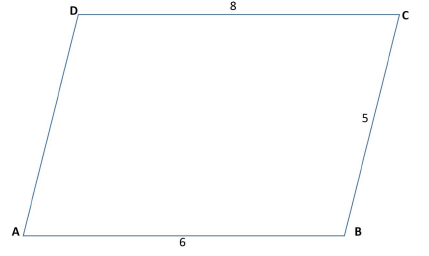In the figure above, ABCD is a quadrilateral in which sides AB and CD are parallel. What is the area of the quadrilateral?
(1) The distance between sides AB and CD is 4 units
(2) The length of side AD is √17 units

Detailed Solution for Test: Rectangles And Squares- 2 - Question 12

Step 1 & 2: Understand Question and Draw Inference

Given:

• In quadrilateral ABCD, AB || CD
• ABCD is a trapezium

To find: Area of quadrilateral ABCD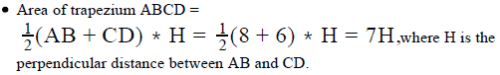So, to find the area of trapezium, we need to find the value of H

Step 3 : Analyze Statement 1 independent

• Statement 1 is:
• H = 4
• This gives the value of H. Hence, Statment 1 is sufficient to find the area.

Step 4 : Analyze Statement 2 independent

• Statement 2 is:
• Let’s drop perpendiculars DE and CF on side AB.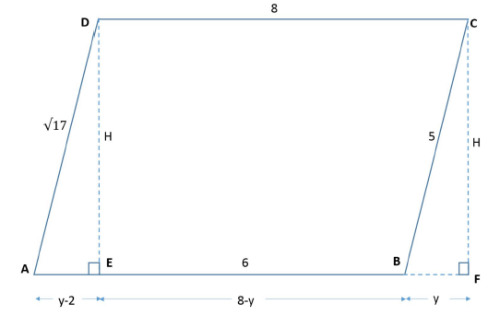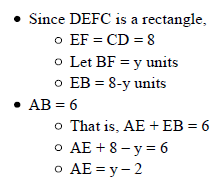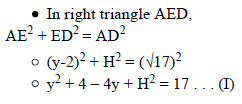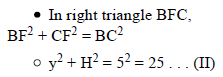• Put (II) in (I):

25 + 4 – 4y = 17

• 4y = 12
• y = 3 . . . (III)

• Put (III) in (II)

32 + H2 = 25

• H2 = 16
• H = 4 (rejecting negative value since length cannot be negative)

• Since we now have a unique value of H, we can find a uniqu e value of the area as well.
• Hence Statement 2 is also sufficient to answer the question

Step 5: Analyze Both Statements Together (if needed)

Since we’ve already arrived at a unique answer in each of Steps 3 and 4, this
step is not required
Therefore, the correct answer is Option D.

Test: Rectangles And Squares- 2 - Question 13

If parallelogram ABCD has a perimeter of 12 units and ∠ADC = 120 , what is the area of the parallelogram?
(1) BD = 2√3 units
(2) The distance between sides AB and CD is √3 units

Detailed Solution for Test: Rectangles And Squares- 2 - Question 13

Step 1 & 2: Understand Question and Draw Inference

Given

• ABCD is a parallelogram.
• Let AB and CD measure p units and AD and BC measure q units
• 2(p + q) = 12
• p + q = 6 . . . (I)
• Using the properties of parallel lines, we can mark the other ∠s of the parallelogram as below: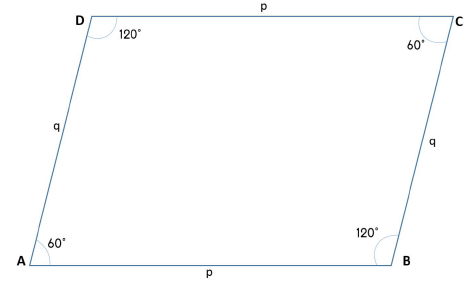To Find : Area of the parallelogram

• Area = p * h, where h is the perpendicular distance between the base and the side opposite to it.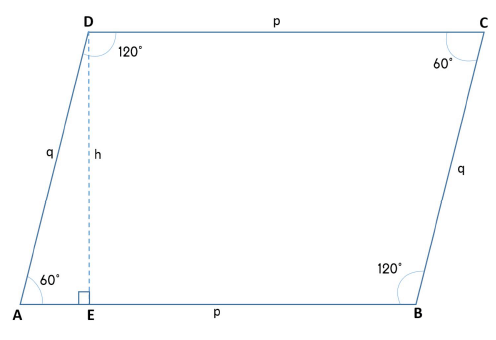• Since ΔAED is a 30-60-90 triangle, applying the angle-side property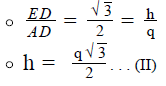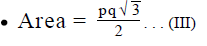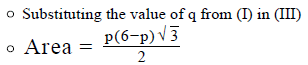Step 3 : Analyze Statement 1 independent

BD = 2√3 units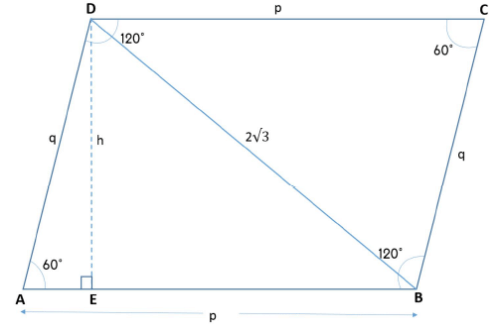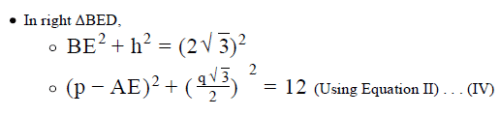• In right ΔAED, applying side ratio property of 30-60-90 triangle, we get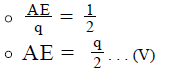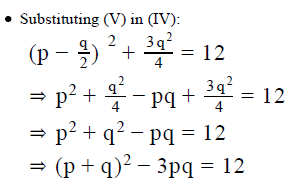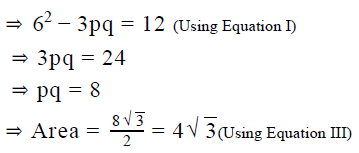• Since we get a unique value of Area, Statement 1 is sufficient to answer

Step 4 : Analyze Statement 2 independent

h= √3 units

• From Equation II, q = 2
• From Equation I, p = 4
• Since we now know the value of p and q, we can find the value of product pq, and hence of the area of the parallelogram, using Equation III
• Hence, even Statement 2 is sufficient to answer the question

Step 5: Analyze Both Statements Together (if needed)

Since we’ve already arrived at a unique answer in Steps 3 and 4 each, this step
is not required
Hence the correct answer is Option D .

Test: Rectangles And Squares- 2 - Question 14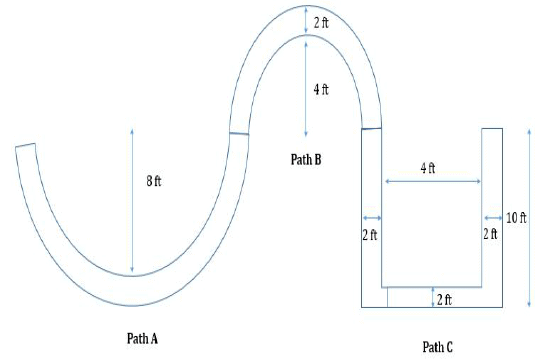The figure above shows the walking path in a park, which is to be covered with tiles. Paths A and path B are semi-circular in shape with the radius of the inner boundary of the path being equal to 8 feet and 4 feet respectively. If the cost of laying the tiles is \$5 per square feet and the path has a uniform width of 2 feet across the park, which of the following is the closest to the amount it will cost, in dollars, to cover the complete walking path with tiles?

Detailed Solution for Test: Rectangles And Squares- 2 - Question 14

Given

• Walking path in the park with its dimensions
• Cost of laying tiles = \$5 per square feet

To Find

• Total cost to lay the tiles on the complete walking path

Approach

• [ Total cost of laying tiles ] = [ Total area of the path in square feet ] * [Cost per square feet ]
• As we are given the cost per square feet, we need to find the total area of the path
• The path consists of two semi-circular regions(path A and path B), two square regions (Regions 2) and three rectangular regions(regions 1 and 3) as shown in the figure below
• Area of a semi-circle =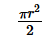• Please note that as paths A and B lie between two semi-circles, we will find the area of the paths by subtracting the areas of the semi-circles, within which the paths lie.
• Area of a square = side
• Area of a rectangle = length * breadth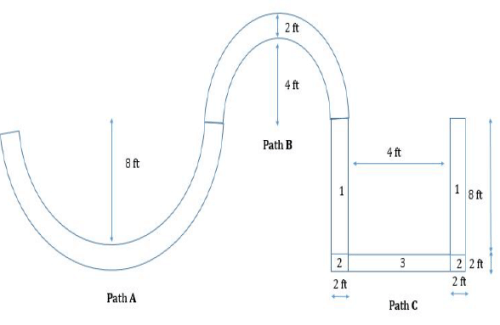• As we are given the dimensions of each of the region, we can find their areas

Working out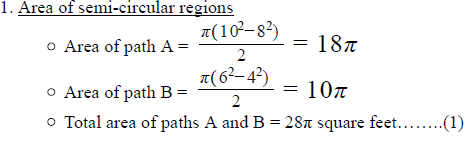2. Area of rectangular regions

• Area of region 1 = 8 *2 = 16
• Area of region 3 = 4*2 = 8
• Total area of rectangular regions = 16 * 2 + 8 = 40 square feet………(2)

3. Area of square region

• Area of region 2 = 2*2 = 4
• Total area of square regions = 4 *2 = 8 square feet………(3)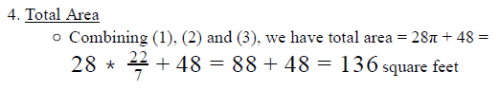5. Total cost of laying tiles = 136 * 5 = \$680
Hence the correct answer is OPTION C

Test: Rectangles And Squares- 2 - Question 15

Is one of the angles of the quadrilateral ABCD equal to 90 degrees?
(1) ABCD is a parallelogram
(2) One of the interior angles of ABCD is equal to 60 degrees

Detailed Solution for Test: Rectangles And Squares- 2 - Question 15

Step 1 & 2: Understand Question and Draw Inference

Given

To Find

• Is one of the angles of quadrilateral ABCD = 90o
• Let the angles be a, b, c and d. Hence a + b + c+ d= 360o

Step 3 : Analyze Statement 1 independent

(1) Statement 1 states that "ABCD is a parallelogram"

• For a parallelogram opposite angles are equal,
• Thus, a = c and b = d. Also, a + d = 180 and b + c = 180
• However, it does not tell us about the value of the angles of the quadrilateral.
• Hence Statement 1 is Insufficient to answer the question.

Step 4 : Analyze Statement 2 independent

(2) Statement 2 states that "One of the interior angles of ABCD is equal to 60 degrees"

• a = 60o
• b + c + d = 300o
• However, this does not tell us about the value of the other 3 angles.
• Hence Statement 2 is also insufficient to answer the question.

Step 5: Analyze Both Statements Together (if needed)

• As a = 60o , c = 60o
• b+ d = 240o
• 2b = 240o
• b=d= 120o
• ​Hence, by combining both the statements we can get the answer.
• Therefore, the correct answer is Option C .
Test: Rectangles And Squares- 2 - Question 16

What is the area of rectangle ABCD?
(1) The length of side AB is 2 units more than the length of side BC.
(2) The length of the diagonal AC is 10 units.

Detailed Solution for Test: Rectangles And Squares- 2 - Question 16

Step 1 & 2: Understand Question and Draw Inference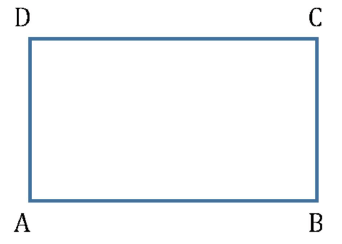Given: Rectangle ABCD

To Find: Area of rectangle ABCD

• = AB*BC

Step 3 : Analyze Statement 1 independent

Statement 1 says that ‘The length of side AB is 2 units more than the length of
side BC'
So, AB = BC + 2

• So, Area of rectangle ABCD = BC*(BC+2)
• However, since we do not know the measure of side BC, we cannot find the area of rectangle ABCD yet

Statement 1 alone is not sufficient to answer the question

Step 4 : Analyze Statement 2 independent

Statement 2 says that 'The length of the diagaonal AC is 10 units'
So, AC = 10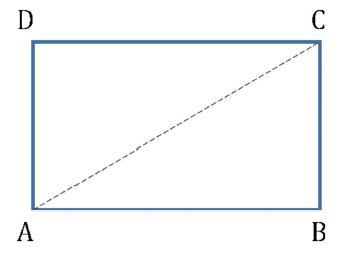•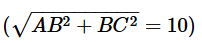• AB2 + BC2 = 100
• This is one quadratic equation with 2 unknowns. Multiple values of AB and BC will satisfy this equation.
• Therefore, Statement 2 doesn’t lead us to unique values of AB and BC, and hence of the product AB*BC
•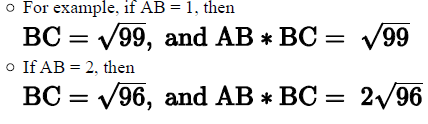• So, Statement 2 alone is not sufficient

Step 5: Analyze Both Statements Together (if needed)

• From Statement 1, AB = BC + 2
• From Statement 2, AB + BC = 100
• Combining the 2 statements: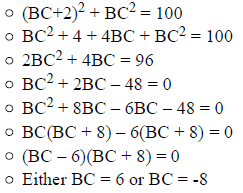• The negative root is rejected because, being a side of a rectangle, the measure of BC cannot be negative
• So, BC = 6
• Therefore, AB = 6 + 2 = 8
• Since we now know the measure of AB and BC, we can find the measure of product AB*BC

So, the 2 statements together are sufficient to answer the question

Test: Rectangles And Squares- 2 - Question 17

In the figure given above, a rectangular photograph is placed in a black frame that has a uniform width of x centimeters between the photograph and the outer boundary of the frame. If the difference between the perimeter of the photograph and of the outer boundary of the frame is 32 centimeters, what is the width in centimeters of the black frame?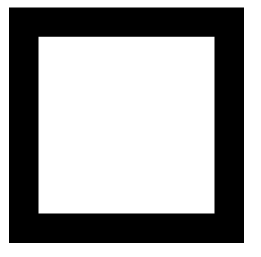Detailed Solution for Test: Rectangles And Squares- 2 - Question 17

Given

• Width of frame = x centimeters
• Perimeter (Outer frame) - Perimeter(photograph) = 32
• Let’s assume the length and breadth of the outer frame be l and b centimeters respectively.

To Find: Value of x?

Approach

1. As we know that x is the distance between the photograph and the outer frame, we can find the dimensions of the photograph in terms of l, b and x.
2. Now, we know that Perimeter of the outer frame = 2(l+b)
1. We will then use the relation of difference in perimeters of the outer frame and the photograph to find the value of x.

Working out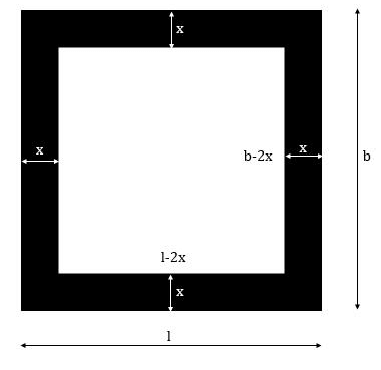1. As the width of the frame is x centimeters, the length of the rectangular photograph = l - x - x = l +2x
1. Similarly, the width of the frame = b - x - x = b -2x.
2. So, perimeter of the rectangular photograph = 2 (l +b -4x) = 2(l + b) - 8x
3. Perimeter (Outer frame) – Perimeter(photograph) = 32
a. 2(l + b)– 2(l + b) + 8x = 32
b. 8x = 32
c. x = 4 centimeters

Hence, the width of the frame is 4 centimeters

Test: Rectangles And Squares- 2 - Question 18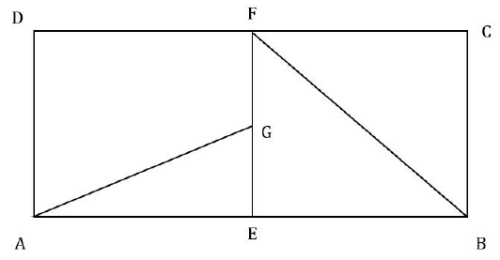In the given figure ABCD is a rectangle and EF || AD. AEG and FEB are two triangles inscribed in the rectangle ABCD such that the area of triangle AEG is half of the area of triangle FEB. What is the area of rectangle ABCD?
(1) EBCF is a square of side length 5 centimeters.
(2) A line passing through point G and parallel to AB divides the rectangle ABCD into 2 equal halves

Detailed Solution for Test: Rectangles And Squares- 2 - Question 18

Step 1 & 2: Understand Question and Draw Inference

• ABCD is a rectangle
• AB = CD and AD = BC
• Area(ΔAEG) = ½ * Area(ΔFEB)
• ½ * AE * EG = ¼ * EB * EF

(Since EF || AD and ABCD is a rectangle, So, Angle(FEB) =90° »

AE is perpendicular to EG and EB is perpendicular to EF)

• AE * EG = ½ * EB * EF

To Find: Area of rectangle ABCD

• Area of ABCD = AB * AD
• Thus to find the area of ABCD, we need to find the actual lengths of AB and AD.

Step 3 : Analyze Statement 1 independent

• Statement 1 states that –
• EBCF is a square of side length 5 centimeters.
• As EBCF is a square, EB = BC =CF = FE = 5 centimeters
• Area of rectangle ABCD = Area of (EBCF +AEFD) = (EB +AE*EF=25+AE*5).
• As we do not know the value of AE, we cannot find the area of rectangle ABCD.
• Hence Statement 1 is insufficient to answer the question

Step 4 : Analyze Statement 2 independent

• Statement 2 states that –
• A line passing through point G and parallel to AB divides the rectangle ABCD into 2 equal halves
• As the line passing through G divides the rectangle into 2 equal halves, the portion of the rectangle above this line segment and below this line segment should be symmetric.
• So, we can write EG = GF
• Also, we know from our question statement analysis that AE * EG = ½ * EB * EF = ½ * EB * 2EG
• So, we have AE = EB

However, we do not know anything about the lengths of the sides of the rectangle. So, we cannot calculate the area of the rectangle ABCD

• Hence Statement 2 is insufficient to answer the question.

Step 5: Analyze Both Statements Together (if needed)

• From statement 1 we inferred –
• Area of rectangle ABCD = 25 + AE * 5, where EB = EB = BC =CF = FE = 5 centimeters
• From statement 2 we inferred –
• AE = EB
• Using both the statements we can infer that
• AE = 5 centimeters.
• Thus, Area of rectangle ABCD = 25 + 5*5 = 50 cm
• Hence by combining both the statement, we can find the area of the
• rectangle and the correct answer is OPTION  C
Test: Rectangles And Squares- 2 - Question 19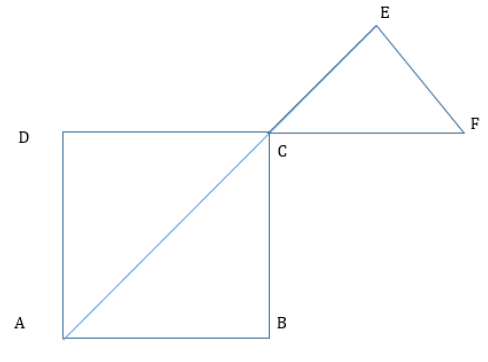In the figure given above, the diagonal AC and side DC of square ABCD are extended to form sides of the isosceles triangle CEF where CE = EF. If the length of side CF is 2√2 and the ratio of areas of the triangle CEF to the square ABCD is 1:8, what is the length of the side of square ABCD?

Detailed Solution for Test: Rectangles And Squares- 2 - Question 19

Given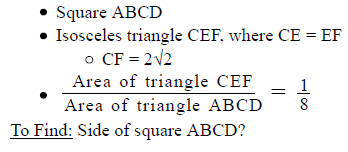Approach

1. We know that area of square ABCD = side . So, if we can find the area of triangle CEF, we can find the area of square ABCD, which will give us the side length of square ABCD.
2. In triangle CEF, we only know the side length CF. So, we need to find the lengths of other sides and the angles of the triangle.
3. Now, we know that diagonals of a square bisects its angles. Also, since AE and DF are straight intersecting lines, ∠ECF = ∠DCA (vertically opposite angles)
1. Since we now know ∠ECF, we can calculate the other angles of the
triangle CEF.

Working out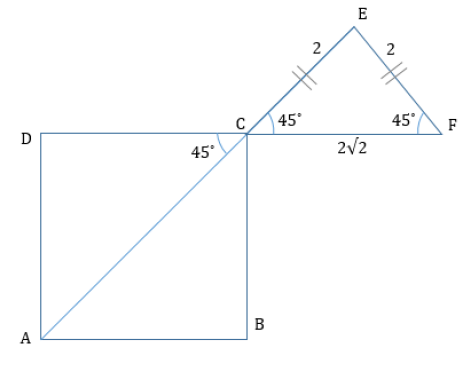1. As the diagonals of the square bisect the vertex angles, ∠DCA = 450
1. Since ∠ECF and ∠DCA are vertically opposite angles, ∠ECF = ∠DCA = 450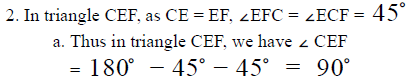3. So, triangle CEF is an isosceles right angled triangle with its hypotenuse equal to 2√2
Using the 45o - 45o  - 90triangle property, we have CE= EF = 2

4. Hence, area of triangle CEF = ½ * CE * EF = ½ * 2* 2 = 2 square centimeters

5. Using the relation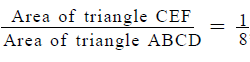we have Ar(ABCD) = 16

6. Thus, side of square ABCD = √16 = 4 centimeters

Test: Rectangles And Squares- 2 - Question 20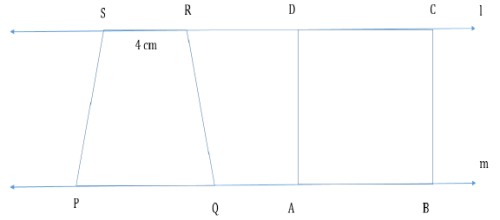Square ABCD and Trapezium PQRS lie between the parallel lines l and m as shown in the figure above. If the area of the square ABCD and trapezium PQRS is equal to 64 square centimeters each, what is the length in centimeters of PQ?

Detailed Solution for Test: Rectangles And Squares- 2 - Question 20

Given

• Parallel lines L and M
• Square ABCD and trapezium PQRS
• RS = 4 centimeters
• Area of square = Area of trapezium = 64 cm

To Find: Length of PQ?

Approach

1. We know that Area of trapezium PQRS = ½ * (PQ + RS)* distance between parallel sides PQ and RS.
1. As we know the length of RS and the area of trapezium PQRS, we need to find the distance between the parallel lines l and m to find the value of PQ
2. The distance between the parallel lines is equal to the side length of the square ABCD.
1. Since we know the area of square ABCD, we can find the distance
between the parallel lines

Working out

1. Area of square ABCD = 64
2. AD = distance between parallel lines = 8
3. So, Area of trapezium PQRS = ½ * (PQ + RS) * distance between parallel lines
a. 64 = ½ *(PQ +4) * 8
b. PQ = 12 centimeters

## Quantitative for GMAT

88 videos|99 docs|165 tests
 Use Code STAYHOME200 and get INR 200 additional OFF Use Coupon Code
Information about Test: Rectangles And Squares- 2 Page
In this test you can find the Exam questions for Test: Rectangles And Squares- 2 solved & explained in the simplest way possible. Besides giving Questions and answers for Test: Rectangles And Squares- 2, EduRev gives you an ample number of Online tests for practice

## Quantitative for GMAT

88 videos|99 docs|165 tests